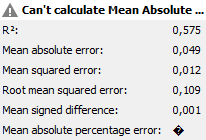# Linear Regression - Prediction Standard Error

Hello,

I´m building a Linear Regression model between 2 variables and after applying the Linear Regression Learner Node, I can obtain the following statisctics:

Variable Coeff. Std. Err. t-value P>|t|
X 0,6935 0,0503 13,7959 0.0
Intercept -0,0099 0,0086 -1,1423 0,2554

Multiple R-Squared: 0,5923

The Standard Errors obtained are for the intercept and variable X, but not for Y (predicted value). Is there a way to obtain this Standard Error?

Thanks.

Hello @garnonay,
you can get that information using the Numeric Scorer node. It is unfortunately not output by the Linear Regression Learner itself.
Kind regards
Alexander

1 Like

Hello @AlexanderFillbrunn,

Can you let me know how? Applying a Numeric Scorer to a previous node with 2 numeric columns (X and Y), I cannot get the Predicted Standard Error of Y as an output from Numeric Scorer.

This is what I get:Thanks.

Hi,
I was under the impression that the standard error is just the mean absolute error on the training data. This is how it is defined here as well:

The standard error of the regression (S), also known as the standard error of the estimate, represents the average distance that the observed values fall from the regression line. Conveniently, it tells you how wrong the regression model is on average using the units of the response variable.

Kind regards
Alexander

1 Like

Hi,

That´s the value I´m looking for, the standard error of the regression, which is different to the mean absolute error…

Is there a way for me to get this in Knime? In Excel there´s a formula for it (STEYX)

Thanks

Hi,
If you look at the formula at https://support.office.com/en-us/article/steyx-function-6ce74b2c-449d-4a6e-b9ac-f9cef5ba48ab, you see that it always contains x and y. This is the error in y under the influence of a certain x. As the link I posted before states, the standard error just for y is the mean absolute error on the training data, not taking into account any particular x. If this is not what you need, please provide the formula you would like to be used for the calculation and we might be able to provide a component for calculating it.
Kind regards
Alexander

This topic was automatically closed 182 days after the last reply. New replies are no longer allowed.